# Integration by Parts - Indefinite Integration JEE Notes | EduRev

## JEE : Integration by Parts - Indefinite Integration JEE Notes | EduRev

The document Integration by Parts - Indefinite Integration JEE Notes | EduRev is a part of the JEE Course Mathematics (Maths) Class 12.
All you need of JEE at this link: JEE

Integration By Parts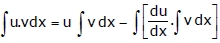dx where u & v are differentiable functions.

Note : While using integration by parts, choose u & v such that
(a)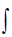dx v is simple &

(b)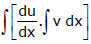dx is simple to integrate.

This is generally obtained, by keeping the order of u & v as per the order of the letter in ILATE, where

I – Inverse function
L – Logarithmic function
A – Algebraic function
T – Trigonometric function
E – Exponential function

Ex.38 Integrate xlog x

Sol.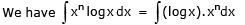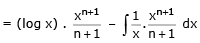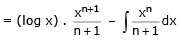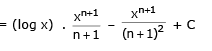Ex.39 Evaluate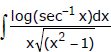dx

Sol.

Put sec–1 x = t so that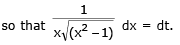Then the given integral  =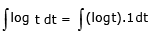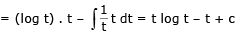= t (log t – log e) + c  = sec–1 x (log (sec–1 x) – 1) + c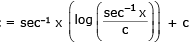Ex.40 Evaluate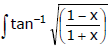dx.

Sol.

Put x = cos θ so that dx = - sin θ dθ. the given integral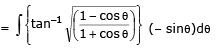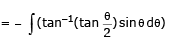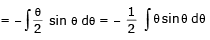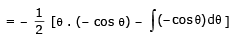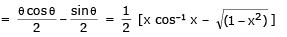Ex.41 Evaluate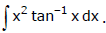Sol.

We have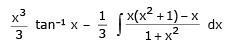[ x3 = x(x2 + 1) - x]

integrating by parts taking x2 as the second function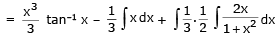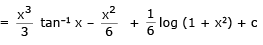Ex.42 Evaluate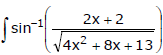dx.

Sol.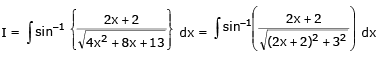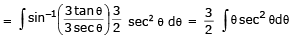(put, 2x + 2 = 3 tanθ ⇒ 2 dx = 3 sec2θ dθ )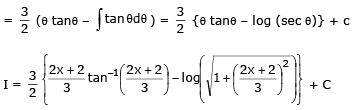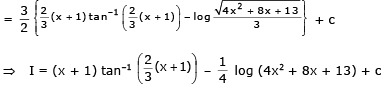Ex.43 If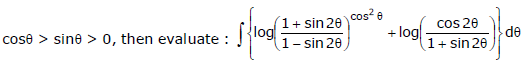Sol.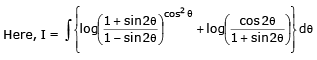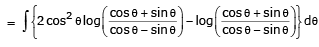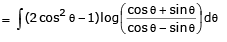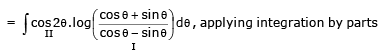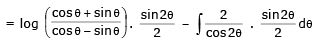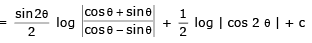REMEMBER THIS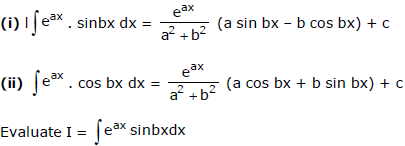Integrating by parts taking sin bx as the second function,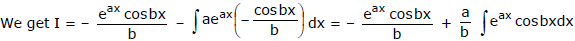Again integrating by parts taking cos bx as the second function, we get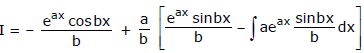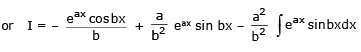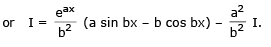Transposing the term -a2/b2 I to the left hand side, we get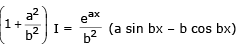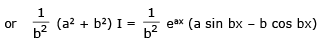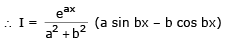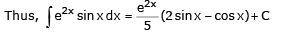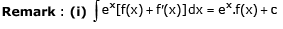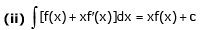Ex.44 Evaluate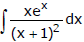Sol.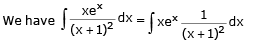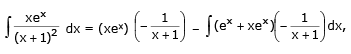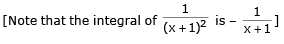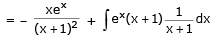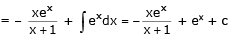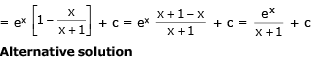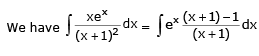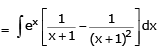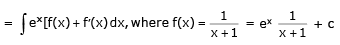Ex.45 Evaluate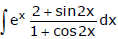Sol.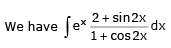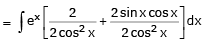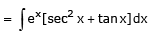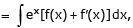x where f(x) = tan x = ex f(x) + c = ex tanx + c

Ex.46 Evaluate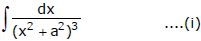Sol.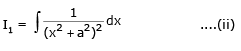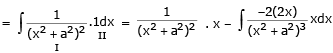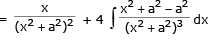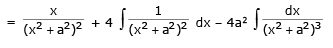using, previous example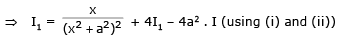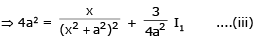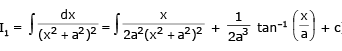Offer running on EduRev: Apply code STAYHOME200 to get INR 200 off on our premium plan EduRev Infinity!

## Mathematics (Maths) Class 12

209 videos|222 docs|124 tests

,

,

,

,

,

,

,

,

,

,

,

,

,

,

,

,

,

,

,

,

,

;